# Exact solutions of Benjamin-Bona-Mahony-Burgers-type nonlinear pseudo-parabolic equations

## Abstract

In this paper, we consider some nonlinear pseudo-parabolic Benjamin-Bona-Mahony-Burgers (BBMB) equations. These equations are of a class of nonlinear pseudo-parabolic or Sobolev-type equations ${u}_{t}-\mathrm{\Delta }{u}_{t}-\alpha \mathrm{\Delta }u=f\left(x,u,\mathrm{\nabla }u\right)$, α is a fixed positive constant, arising from the mathematical physics. The tanh method with the aid of symbolic computational system is employed to investigate exact solutions of BBMB-type equations and the exact solutions are found. The results obtained can be viewed as verification and improvement of the previously known data.

## 1 Introduction

The partial differential equations of the form

${u}_{t}-\eta \mathrm{\Delta }{u}_{t}-\alpha \mathrm{\Delta }u=f\left(x,u,\mathrm{\nabla }u\right)$
(1)

arise in many areas of mathematics and physics, where $u=u\left(x,t\right)$, $x\in \mathrm{\Omega }\subset {\mathbb{R}}^{n}$, $t\ge 0$, η and α are non-negative constants, Δ denotes the Laplace operator acting on the space variables x. Equations of type (1) with only one time derivative appearing in the highest-order term are called pseudo-parabolic and they are a special case of Sobolev equations. They are characterized by derivatives of mixed type (i.e., time and space derivatives together) appearing in the highest-order terms of the equation and were studied by Sobolev . Sobolev equations have been used to describe many physical phenomena . Equation (1) arises as a mathematical model for the unidirectional propagation of nonlinear, dispersive, long waves. In applications, u is typically the amplitude or velocity, x is proportional to the distance in the direction of propagation, and t is proportional to elapsed time .

An important special case of (1) is the Benjamin-Bona-Mahony-Burgers (BBMB) equation

$-{u}_{xxt}+{u}_{t}-\alpha {u}_{xx}+\left(1+u\right){u}_{x}=0.$
(2)

It has been proposed in  as a model to study the unidirectional long waves of small amplitudes in water, which is an alternative to the Korteweg-de Vries equation of the form

${u}_{xxx}+{u}_{t}-{u}_{xx}+u{u}_{x}=0.$
(3)

The BBMB equation has been tackled and investigated by many authors. For more details, we refer the reader to  and the references therein.

In , a generalized Benjamin-Bona-Mahony-Burgers equation

$-{u}_{xxt}+{u}_{t}-\alpha {u}_{xx}+\beta {u}_{x}+{\left(g\left(u\right)\right)}_{x}=0$
(4)

has been considered and a set of new solitons, kinks, antikinks, compactons, and Wadati solitons have been derived using by the classical Lie method, where α is a positive constant, $\beta \in \mathbb{R}$, and $g\left(u\right)$ is a ${C}^{2}$-smooth nonlinear function. Equation (4) with the dissipative term $\alpha {u}_{xx}$ arises in the phenomena for both the bore propagation and the water waves.

Peregrine  and Benjamin, Bona, and Mahony  have proposed equation (4) with the parameters $g\left(u\right)=u{u}_{x}$, $\alpha =0$, and $\beta =1$. Furthermore, Benjamin, Bona, and Mahony proposed equation (4) as an alternative regularized long-wave equation with the same parameters.

Khaled, Momani, and Alawneh obtained explicit and numerical solutions of BBMB equation (4) by using the Adomian’s decomposition method  .

Tari and Ganji implemented variational iteration and homotopy perturbation methods obtaining approximate explicit solutions for (4) with $g\left(u\right)=\frac{{u}^{2}}{2}$  and El-Wakil, Abdou, and Hendi used another method (the exp-function) to obtain the generalized solitary solutions and periodic solutions of this equation .

In addition, we consider $g\left(u\right)=\frac{{u}^{3}}{3}$ and obtain analytic solutions in a closed form.

The aim of this work is twofold. First, it is to obtain the exact solutions of the Benjamin-Bona-Mahony-Burgers (BBMB) equation and the generalized Benjamin-Bona-Mahony-Burgers equation with $g\left(u\right)=u{u}_{x}$, $g\left(u\right)=\frac{{u}^{2}}{2}$, $g\left(u\right)=\frac{{u}^{3}}{3}$; and second, it is to show that the tanh method can be applied to obtain the solutions of pseudo-parabolic equations.

## 2 Outline of the tanh method

Wazwaz has summarized the tanh method  in the following manner:

1. (i)

First, consider a general form of the nonlinear equation

$P\left(u,{u}_{t},{u}_{x},{u}_{xx},\dots \right)=0.$
(5)
2. (ii)

To find the traveling wave solution of equation (5), the wave variable $\xi =x-Vt$ is introduced so that

$u\left(x,t\right)=U\left(\mu \xi \right).$
(6)

Based on this, one may use the following changes:

$\begin{array}{r}\frac{\partial }{\partial t}=-V\frac{d}{d\xi },\\ \frac{\partial }{\partial x}=\mu \frac{d}{d\xi },\\ \frac{{\partial }^{2}}{\partial {x}^{2}}={\mu }^{2}\frac{{d}^{2}}{d{\xi }^{2}},\\ \frac{{\partial }^{3}}{\partial {x}^{3}}={\mu }^{3}\frac{{d}^{3}}{d{\xi }^{3}}\end{array}$
(7)

and so on for other derivatives. Using (7) changes PDE (5) to an ODE

$Q\left(U,{U}^{\mathrm{\prime }},{U}^{\mathrm{\prime }\mathrm{\prime }},\dots \right)=0.$
(8)
1. (iii)

If all terms of the resulting ODE contain derivatives in ξ, then by integrating this equation and by considering the constant of integration to be zero, one obtains a simplified ODE.

2. (iv)

A new independent variable

$Y=tanh\left(\mu \xi \right)$
(9)

is introduced that leads to the change of derivatives: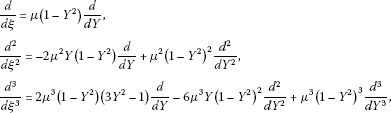(10)

where other derivatives can be derived in a similar manner.

1. (v)

The ansatz of the form

$U\left(\mu \xi \right)=S\left(Y\right)=\sum _{k=0}^{M}{a}_{k}{Y}^{k}+\sum _{k=1}^{M}{b}_{k}{Y}^{-k}$
(11)

is introduced, where M is a positive integer, in most cases, that will be determined. If M is not an integer, then a transformation formula is used to overcome this difficulty. Substituting (10) and (11) into ODE (8) yields an equation in powers of Y.

1. (vi)

To determine the parameter M, the linear terms of highest order in the resulting equation with the highest-order nonlinear terms are balanced. With M determined, one collects all the coefficients of powers of Y in the resulting equation where these coefficients have to vanish. This will give a system of algebraic equations involving the ${a}_{k}$ and ${b}_{k}$ ($k=0,\dots ,M$), V, and μ. Having determined these parameters, knowing that M is a positive integer in most cases, and using (11), one obtains an analytic solution in a closed form.

Throughout the work, Mathematica or Maple is used to deal with the tedious algebraic operations.

## 3 The Benjamin-Bona-Mahony-Burgers (BBMB) equation

The Benjamin-Bona-Mahony-Burgers (BBMB) equation is given by

$-{u}_{xxt}+{u}_{t}-\alpha {u}_{xx}+\left(1+u\right){u}_{x}=0,$
(12)

where α is a positive constant. Using the wave variable $\xi =x-Vt$ carries (12) into the ODE

$\left(-V+1\right)U+V{U}^{\mathrm{\prime }\mathrm{\prime }}-\alpha {U}^{\mathrm{\prime }}+\frac{1}{2}{U}^{2}=0.$
(13)

Balancing ${U}^{2}$ with ${U}^{\mathrm{\prime }\mathrm{\prime }}$ in (13) gives $M=2$. The tanh method admits the use of the finite expansion

$U\left(\xi \right)=S\left(Y\right)=\sum _{k=0}^{2}{a}_{k}{Y}^{k}+\sum _{k=1}^{2}{b}_{k}{Y}^{-k},$
(14)

where $Y=tanh\left(\mu \xi \right)$. Substituting (14) into (13), collecting the coefficients of Y, and setting it equal to zero, we find the system of equations

$\begin{array}{r}{Y}^{8}:\phantom{\rule{1em}{0ex}}12V{\mu }^{2}{a}_{2}+{a}_{2}^{2}=0,\\ {Y}^{7}:\phantom{\rule{1em}{0ex}}4V{a}_{1}{\mu }^{2}+4\alpha {a}_{2}\mu +2{a}_{1}{a}_{2}=0,\\ {Y}^{6}:\phantom{\rule{1em}{0ex}}-16V{a}_{2}{\mu }^{2}+2\alpha \mu {a}_{1}+{a}_{1}^{2}+2{a}_{2}-2V{a}_{2}+2{a}_{0}{a}_{2}=0,\\ {Y}^{5}:\phantom{\rule{1em}{0ex}}-4V{a}_{1}{\mu }^{2}-4\alpha {a}_{2}\mu +2{a}_{1}-2V{a}_{1}+2{b}_{1}{a}_{2}+2{a}_{0}{a}_{1}=0,\\ {Y}^{4}:\phantom{\rule{1em}{0ex}}2{a}_{0}-2V{a}_{0}+2{b}_{1}{a}_{1}+2{b}_{2}{a}_{2}+{a}_{0}^{2}\\ \phantom{{Y}^{4}:\phantom{\rule{1em}{0ex}}}\phantom{\rule{1em}{0ex}}+4V{\mu }^{2}{b}_{2}+4V{\mu }^{2}{a}_{2}-2\alpha \mu {b}_{1}-2\alpha \mu {a}_{1}=0,\\ {Y}^{3}:\phantom{\rule{1em}{0ex}}-4V{b}_{1}{\mu }^{2}-4\alpha {b}_{2}\mu +2{b}_{1}-2V{b}_{1}+2{b}_{1}{a}_{0}+2{b}_{2}{a}_{1}=0,\\ {Y}^{2}:\phantom{\rule{1em}{0ex}}-16V{b}_{2}{\mu }^{2}+2\alpha \mu {b}_{1}+{b}_{1}^{2}+2{b}_{2}-2V{b}_{2}+2{b}_{2}{a}_{0}=0,\\ {Y}^{1}:\phantom{\rule{1em}{0ex}}4V{b}_{1}{\mu }^{2}+4\alpha {b}_{2}\mu +2{b}_{1}{b}_{2}=0,\\ {Y}^{0}:\phantom{\rule{1em}{0ex}}{b}_{2}^{2}+12V{\mu }^{2}{b}_{2}=0.\end{array}$
(15)

Using Maple gives nine sets of solutions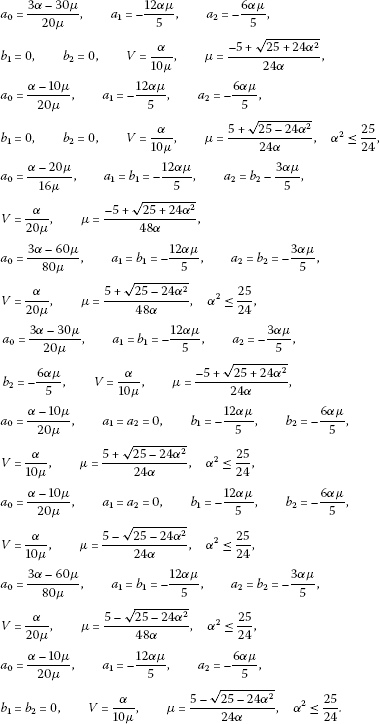(16)

These sets give the following solutions respectively: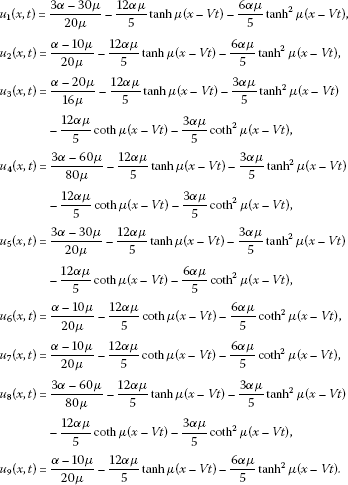(17)

If we accept $\alpha =1$, then we obtain solutions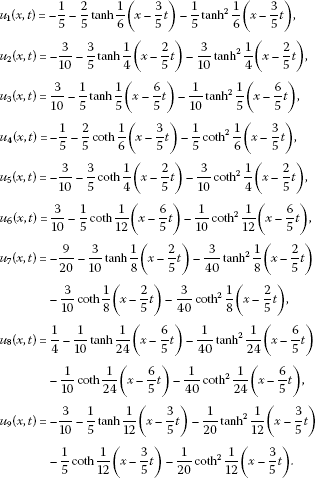(18)

## 4 The generalized Benjamin-Bona-Mahony-Burgers equation

We consider the generalized Benjamin-Bona-Mahony-Burgers equation

$-{u}_{xxt}+{u}_{t}-\alpha {u}_{xx}+\beta {u}_{x}+{\left(g\left(u\right)\right)}_{x}=0,$
(19)

where α is a positive constant and $\beta \in \mathbb{R}$.

Case 1. $g\left(u\right)=u{u}_{x}$.

Using the wave variable $\xi =x-Vt$ carries (19) into the ODE

$-VU+V{U}^{\mathrm{\prime }\mathrm{\prime }}-\alpha {U}^{\mathrm{\prime }}+\beta U+U{U}^{\mathrm{\prime }}=0.$
(20)

Balancing ${U}^{\mathrm{\prime }}$ with $U{U}^{\mathrm{\prime }}$ in (20) gives $M=1$. Using the finite expansion

$U\left(\xi \right)=S\left(Y\right)=\sum _{k=0}^{1}{a}_{k}{Y}^{k}+\sum _{k=1}^{1}{b}_{k}{Y}^{-k},$
(21)

we find the system of equations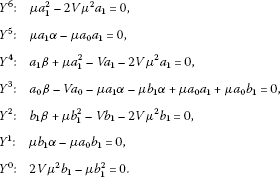(22)

Maple gives three sets of solutions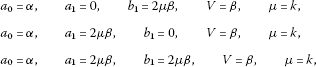(23)

where k is left as a free parameter. These give the following solutions: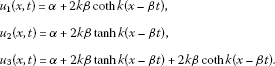(24)

Case 2. $g\left(u\right)=\frac{{u}^{2}}{2}$.

Using the wave variable $\xi =x-Vt$, then by integrating this equation and considering the constant of integration to be zero, we obtain

$\left(V-\beta \right)U-V{U}^{\mathrm{\prime }\mathrm{\prime }}+\alpha {U}^{\mathrm{\prime }}+\frac{1}{2}{U}^{2}=0.$
(25)

Balancing the second term with the last term in (25) gives $M=2$. Using the finite expansion

$U\left(\xi \right)=S\left(Y\right)=\sum _{k=0}^{2}{a}_{k}{Y}^{k}+\sum _{k=1}^{2}{b}_{k}{Y}^{-k},$
(26)

we find the system of equations

$\begin{array}{r}{Y}^{8}:\phantom{\rule{1em}{0ex}}{a}_{2}^{2}-12V{\mu }^{2}{a}_{2}=0,\\ {Y}^{7}:\phantom{\rule{1em}{0ex}}4V{a}_{1}{\mu }^{2}+4\alpha {a}_{2}\mu -2{a}_{1}{a}_{2}=0,\\ {Y}^{6}:\phantom{\rule{1em}{0ex}}16V{a}_{2}{\mu }^{2}-2\alpha \mu {a}_{1}+{a}_{1}^{2}+2V{a}_{2}-2\beta {a}_{2}+2{a}_{0}{a}_{2}=0,\\ {Y}^{5}:\phantom{\rule{1em}{0ex}}4V{a}_{1}{\mu }^{2}+4\alpha {a}_{2}\mu +2V{a}_{1}-2\beta {a}_{1}+2{b}_{1}{a}_{2}+2{a}_{0}{a}_{1}=0,\\ {Y}^{4}:\phantom{\rule{1em}{0ex}}2V{a}_{0}-2\beta {a}_{0}+2{b}_{1}{a}_{1}+2{b}_{2}{a}_{2}+{a}_{0}^{2}\\ \phantom{{Y}^{4}:\phantom{\rule{1em}{0ex}}}\phantom{\rule{1em}{0ex}}-4V{\mu }^{2}{b}_{2}-4V{\mu }^{2}{a}_{2}+2\alpha \mu {b}_{1}+2\alpha \mu {a}_{1}=0,\\ {Y}^{3}:\phantom{\rule{1em}{0ex}}4V{b}_{1}{\mu }^{2}+4\alpha {b}_{2}\mu +2V{b}_{1}-2\beta {b}_{1}+2{b}_{1}{a}_{0}+2{b}_{2}{a}_{1}=0,\\ {Y}^{2}:\phantom{\rule{1em}{0ex}}16V{b}_{2}{\mu }^{2}-2\alpha \mu {b}_{1}+{b}_{1}^{2}+2V{b}_{2}-2\beta {b}_{2}+2{b}_{2}{a}_{0}=0,\\ {Y}^{1}:\phantom{\rule{1em}{0ex}}4V{b}_{1}{\mu }^{2}+4\alpha {b}_{2}\mu -2{b}_{1}{b}_{2}=0,\\ {Y}^{0}:\phantom{\rule{1em}{0ex}}{b}_{2}^{2}-12V{\mu }^{2}{b}_{2}=0.\end{array}$
(27)

Using Maple, we obtain nine sets of solutions(28)

These sets give the solutions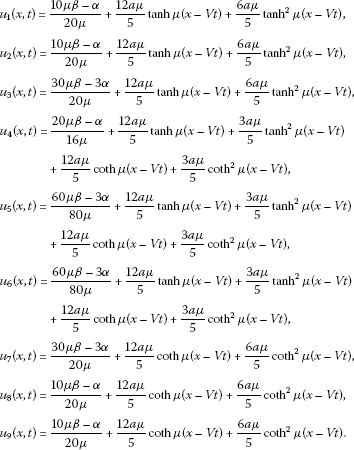(29)

Case 3. $g\left(u\right)=\frac{{u}^{3}}{3}$.

Using the wave variable $\xi =x-Vt$, then by integrating this equation once and considering the constant of integration to be zero, we obtain

$-3VU+3V{U}^{\mathrm{\prime }\mathrm{\prime }}-3\alpha {U}^{\mathrm{\prime }}+3\beta U+{U}^{3}=0.$
(30)

Balancing ${U}^{\mathrm{\prime }\mathrm{\prime }}$ with ${U}^{3}$ in (30) gives $M=1$. Using the finite expansion

$U\left(\xi \right)=S\left(Y\right)=\sum _{k=0}^{1}{a}_{k}{Y}^{k}+\sum _{k=1}^{1}{b}_{k}{Y}^{-k},$
(31)

we find the system of equations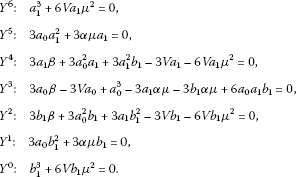(32)

Solving the resulting system, we find the following sets of solutions with $\frac{{\alpha }^{2}}{{\beta }^{2}}\le \frac{9}{8}$: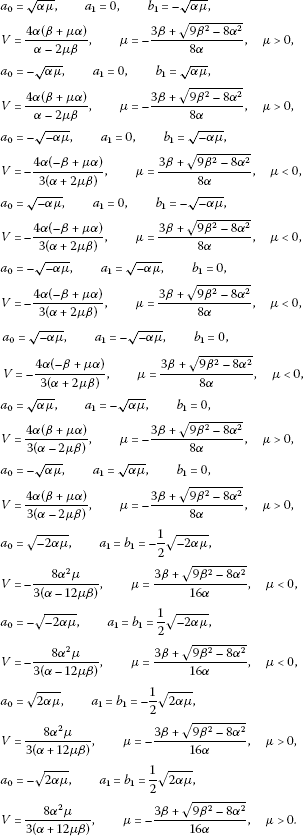(33)

These in turn give the solutions

$\begin{array}{r}{u}_{1}\left(x,t\right)=\sqrt{\alpha \mu }-\sqrt{\alpha \mu }coth\mu \left(x-Vt\right),\\ {u}_{2}\left(x,t\right)=-\sqrt{\alpha \mu }+\sqrt{\alpha \mu }coth\mu \left(x-Vt\right),\\ {u}_{3}\left(x,t\right)=-\sqrt{-\alpha \mu }+\sqrt{-\alpha \mu }coth\mu \left(x-Vt\right),\\ {u}_{4}\left(x,t\right)=\sqrt{-\alpha \mu }-\sqrt{-\alpha \mu }coth\mu \left(x-Vt\right),\\ {u}_{5}\left(x,t\right)=-\sqrt{-\alpha \mu }+\sqrt{-\alpha \mu }tanh\mu \left(x-Vt\right),\\ {u}_{6}\left(x,t\right)=\sqrt{-\alpha \mu }-\sqrt{-\alpha \mu }tanh\mu \left(x-Vt\right),\\ {u}_{7}\left(x,t\right)=\sqrt{-\alpha \mu }-\sqrt{-\alpha \mu }tanh\mu \left(x-Vt\right),\\ {u}_{8}\left(x,t\right)=-\sqrt{-\alpha \mu }+\sqrt{-\alpha \mu }tanh\mu \left(x-Vt\right),\\ {u}_{9}\left(x,t\right)=\sqrt{-2\alpha \mu }-\frac{1}{2}\sqrt{-2\alpha \mu }tanh\mu \left(x-Vt\right)-\frac{1}{2}\sqrt{-2\alpha \mu }coth\mu \left(x-Vt\right),\\ {u}_{10}\left(x,t\right)=-\sqrt{-2\alpha \mu }+\frac{1}{2}\sqrt{-2\alpha \mu }tanh\mu \left(x-Vt\right)+\frac{1}{2}\sqrt{-2\alpha \mu }coth\mu \left(x-Vt\right),\\ {u}_{11}\left(x,t\right)=\sqrt{2\alpha \mu }-\frac{1}{2}\sqrt{2\alpha \mu }tanh\mu \left(x-Vt\right)-\frac{1}{2}\sqrt{2\alpha \mu }coth\mu \left(x-Vt\right),\\ {u}_{12}\left(x,t\right)=-\sqrt{2\alpha \mu }+\frac{1}{2}\sqrt{2\alpha \mu }tanh\mu \left(x-Vt\right)+\frac{1}{2}\sqrt{2\alpha \mu }coth\mu \left(x-Vt\right).\end{array}$
(34)

## 5 Conclusion

In summary, we implemented the tanh method to solve some nonlinear pseudo-parabolic Benjamin-Bona-Mahony-Burgers equations and obtained new solutions which could not be attained in the past. Besides, we have seen that the tanh method is easy to apply and reliable to solve the pseudo-parabolic and the Sobolev-type equations.

## References

1. Sobolev SL: Some new problems in mathematical physics. Izv. Akad. Nauk SSSR, Ser. Mat. 1954, 18: 3-50.

2. Chen PJ, Gurtin ME: On a theory of heat conduction involving two temperatures. Z. Angew. Math. Phys. 1968, 19: 614-627. 10.1007/BF01594969

3. Barenblat G, Zheltov I, Kochina I: Basic concepts in the theory of seepage of homogeneous liquids in fissured rocks. J. Appl. Math. Mech. 1960, 24: 1286-1303. 10.1016/0021-8928(60)90107-6

4. Taylor D: Research of Consolidation of Clays. Massachusetts Institute of Technology Press, Cambridge; 1952.

5. Coleman BD, Noll W: An approximation theorem for functionals with applications to continuum mechanics. Arch. Ration. Mech. Anal. 1960, 6: 355-370. 10.1007/BF00276168

6. Huilgol R: A second order fluid of the differential type. Int. J. Non-Linear Mech. 1968, 3: 471-482. 10.1016/0020-7462(68)90032-2

7. Ting TW: Certain nonsteady flows of second-order fluids. Arch. Ration. Mech. Anal. 1963, 14: 1-26.

8. Barenblat GI, Zheltov IP, Kochina IN: Basic concepts in the theory of seepage of homogeneous liquids in fissured rocks. J. Appl. Math. Mech. 1960, 24: 1286-1303. 10.1016/0021-8928(60)90107-6

9. Karch G: Asymptotic behaviour of solutions to some pseudoparabolic equations. Math. Methods Appl. Sci. 1997, 20: 271-289. 10.1002/(SICI)1099-1476(199702)20:3<271::AID-MMA859>3.0.CO;2-F

10. Benjamin TB, Bona JL, Mahony JJ: Model equations for long waves in nonlinear dispersive systems. Philos. Trans. R. Soc. Lond. Ser. A 1972, 272: 47-78. 10.1098/rsta.1972.0032

11. Raupp MA: Galerkin methods applied to the Benjamin-Bona-Mahony equation. Bull. Braz. Math. Soc. 1975, 6: 65-77. 10.1007/BF02584873

12. Wahlbin L: Error estimates for a Galerkin method for a class of model equations for long waves. Numer. Math. 1975, 23: 289-303.

13. Ewing RE: Time-stepping Galerkin methods for nonlinear Sobolev partial differential equation. SIAM J. Numer. Anal. 1978, 15: 1125-1150. 10.1137/0715075

14. Arnold DN, Douglas J Jr., Thomée V: Superconvergence of finite element approximation to the solution of a Sobolev equation in a single space variable. Math. Comput. 1981, 27: 737-743.

15. Manickam SAV, Pani AK, Chang SK: A second-order splitting combined with orthogonal cubic spline collocation method for the Roseneau equation. Numer. Methods Partial Differ. Equ. 1998, 14: 695-716. 10.1002/(SICI)1098-2426(199811)14:6<695::AID-NUM1>3.0.CO;2-L

16. Bruzon MS, Gandarias ML: Travelling wave solutions for a generalized benjamin-bona-mahony-burgers equation. Int. J. Math. Models Methods Appl. Sci. 2008, 2: 103-108.

17. Peregrine DH: Calculations of the development of an undular bore. J. Fluid Mech. 1996, 25: 321-330.

18. Al-Khaled K, Momani S, Alawneh A: Approximate wave solutions for generalized Benjamin-Bona-Mahony-Burgers equations. Appl. Math. Comput. 2005, 171: 281-292. 10.1016/j.amc.2005.01.056

19. Tari H, Ganji DD: Approximate explicit solutions of nonlinear BBMB equations by He’s methods and comparison with the exact solution. Phys. Lett. A 2007, 367: 95-101. 10.1016/j.physleta.2007.02.085

20. El-Wakil SA, Abdou MA, Hendi A: New periodic wave solutions via Exp-function method. Phys. Lett. A 2008, 372: 830-840. 10.1016/j.physleta.2007.08.033

21. Wazwaz AM: The Hirota’s bilinear method and the tanh-coth method for multiple-soliton solutions of the Sawada-Kotera-Kadomtsev-Petviashvili equation. Appl. Math. Comput. 2008, 200: 160-166. 10.1016/j.amc.2007.11.001

## Author information

Authors

### Corresponding author

Correspondence to Ömer Faruk Gözükızıl.

### Competing interests

The authors declare that they have no competing interests.

### Authors’ contributions

All authors read and approved the final manuscript.

## Rights and permissions

Reprints and Permissions

Gözükızıl, Ö.F., Akçağıl, Ş. Exact solutions of Benjamin-Bona-Mahony-Burgers-type nonlinear pseudo-parabolic equations. Bound Value Probl 2012, 144 (2012). https://doi.org/10.1186/1687-2770-2012-144

• Accepted:

• Published:

• DOI: https://doi.org/10.1186/1687-2770-2012-144

### Keywords

• nonlinear pseudo-parabolic equation
• Benjamin-Bona-Mahony-Burgers (BBMB)-type equation
• Sobolev-type equation
• tanh method